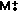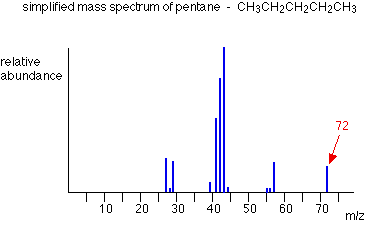MASS SPECTRA - THE MOLECULAR ION (M+) PEAK

```
```

This page explains how to find the relative formula mass (relative molecular mass) of an organic compound from its mass spectrum. It also shows how high resolution mass spectra can be used to find the molecular formula for a compound.

```
```

Using a mass spectrum to find relative formula mass

The formation of molecular ions

When the vaporised organic sample passes into the ionisation chamber of a mass spectrometer, it is bombarded by a stream of electrons. These electrons have a high enough energy to knock an electron off an organic molecule to form a positive ion. This ion is called the molecular ion.

Note:  If you aren't sure about how a mass spectrum is produced, it might be worth taking a quick look at the page describing how a mass spectrometer works.

The molecular ion is often given the symbol M+ or- the dot in this second version represents the fact that somewhere in the ion there will be a single unpaired electron. That's one half of what was originally a pair of electrons - the other half is the electron which was removed in the ionisation process.

The molecular ions tend to be unstable and some of them break into smaller fragments. These fragments produce the familiar stick diagram. Fragmentation is irrelevant to what we are talking about on this page - all we're interested in is the molecular ion.

Note:  If you are interested in a detailed look at fragmentation patterns you could follow this link.

Using the molecular ion to find the relative formula mass

In the mass spectrum, the heaviest ion (the one with the greatest m/z value) is likely to be the molecular ion. A few compounds have mass spectra which don't contain a molecular ion peak, because all the molecular ions break into fragments. That isn't a problem you are likely to meet at A'level.

For example, in the mass spectrum of pentane, the heaviest ion has an m/z value of 72.Note:  This mass spectrum has been drawn using data from the Spectral Data Base System for Organic Compounds (SDBS) at the National Institute of Materials and Chemical Research in Japan.

It has been simplified by omitting all the minor lines with peak heights of 2% or less of the base peak (the tallest peak).

Because the largest m/z value is 72, that represents the largest ion going through the mass spectrometer - and you can reasonably assume that this is the molecular ion. The relative formula mass of the compound is therefore 72.

Note:  This assumes that the charge on the ion is 1+. That's always the case when you are interpreting these mass spectra.

Finding the relative formula mass (relative molecular mass) from a mass spectrum is therefore trivial. Look for the peak with the highest value for m/z, and that value is the relative formula mass of the compound.

There are, however, complications which arise because of the possibility of different isotopes (either of carbon or of chlorine or bromine) in the molecular ion. These cases are dealt with on separate pages.

Note:  The presence of the carbon-13 isotope in a molecular ion causes a little peak 1 unit to the right of the M+ peak. This is called the M+1 peak.

The presence of a chlorine atom in a compound causes two peaks in the molecular ion region - the M+ peak and the M+2 peak depending on whether the particular molecular ion contains a chlorine-35 or chlorine-37 isotope. Bromine creates a similar problem. Follow these links if you are interested - or explore them later via the mass spectrometry menu.

```
```

Using a mass spectrum to find a molecular formula

So far we've been looking at m/z values in a mass spectrum as whole numbers, but it's possible to get far more accurate results using a high resolution mass spectrometer. You can use that more accurate information about the mass of the molecular ion to work out the molecular formula of the compound.

Accurate isotopic masses

For normal calculation purposes, you tend to use rounded-off relative isotopic masses. For example, you are familiar with the numbers:

 1H1 12C12 14N14 16O16

To 4 decimal places, however, these are the relative isotopic masses:

 1H1.0078 12C12 14N14.0031 16O15.9949

The carbon value is 12.0000, of course, because all the other masses are measured on the carbon-12 scale which is based on the carbon-12 isotope having a mass of exactly 12.

```
```

Using these accurate values to find a molecular formula

Two simple organic compounds have a relative formula mass of 44 - propane, C3H8, and ethanal, CH3CHO. Using a high resolution mass spectrometer, you could easily decide which of these you had.

On a high resolution mass spectrometer, the molecular ion peaks for the two compounds give the following m/z values:

 C3H844.0624 CH3CHO44.0261

You can easily check that by adding up numbers from the table of accurate relative isotopic masses above.

A possible exam question

A gas was known to contain only elements from the following list:

 1H1.0078 12C12 14N14.0031 16O15.9949

The gas had a molecular ion peak at m/z = 28.0312 in a high resolution mass spectrometer. What was the gas?

After a bit of playing around, you might reasonably come up with 3 gases which had relative formula masses of approximately 28 and which contained the elements from the list. They are N2, CO and C2H4.

Working out their accurate relative formula masses gives:

 N228.0062 CO27.9949 C2H428.0312

The gas is obviously C2H4.

In an exam, you would hope that - apart from the most simple cases - you would be given the possible formulae to work from. Trying to work out all the possible things which might add up to the value you want is quite time-consuming - and it's easy to miss an important possibility!

```
```
 Questions to test your understanding If this is the first set of questions you have done, please read the introductory page before you start. You will need to use the BACK BUTTON on your browser to come back here afterwards. questions on the molecular ion peak answers
```
```

Where would you like to go now?

To the mass spectrometry menu . . .

To the instrumental analysis menu . . .

To Main Menu . . .

```
```

© Jim Clark 2000 (modified August 2014)